## Friday, November 23, 2007

### Irodov Problem 1.93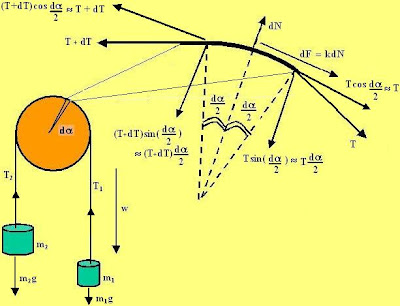The very basic difference between this problem and all other that while in all previous problems there was no friction between the pulley and the string, here there is some friction between the pulley and the thread. So in all the previous problems the thread would simply slip over the pulley and there was no force of friction hindering this slippage. In this problem we have to consider the effect of force of friction between the pulley and the string. One big difference when there is some friction between the string and the pulley is that the tension in different parts of the string will be different and not the same everywhere. The reason is that the tension has to provide for the friction force in addition to the gravity acting on the masses. To understand how the force of friction acts on the string consider an infinitesimally small segment of the string as shown in the figure.

We consider an infinitesimally small part of the string that subtends an angle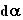at the center of the pulley. The section is shown magnified in the figure and depicts the various forces acting on the piece of the string. There four forces acting on the piece of string , i) the force T with which the rest of the string on the right pulls this string piece, ii) the force T+dT with which the rest of the string on the left, iii) the force of friction dF acting on the string that opposes its slippage and iv) the normal reaction dN that acts normal to the pulley. The tension will keep continuously increasing along the direction of mass m2 since friction always acts in a direction so as to oppose the slippage as shown in the figure. Let us consider forces acting in the tangential and radial directions.

Along the normal direction we have three forces acting, i) the components of tension pulling this string piece along the radial direction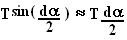and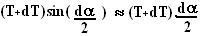as shown in the figure ( for small values of,can be approximated bysee here ) and ii) the normal reaction dN acting upwards. Since the piece of string does not have any component of acceleration in the radial direction we have,As the limits of all the variables dN, dT andtend to 0, the term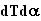will tend to 0 and equation (1) will be reduced to,Now let us consider forces acting on the string in the tangential direction. There are three forces acting along this direction, i) the component of tension acting on the left side of the string, ii) the component acting on the right of the stringand iii) the force of friction dF as shown in the figure. At the slipping point, dF = kdN . The string has no mass and the system has just begun to accelerate. Thus we have,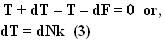From (3) and (2) we can write,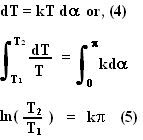a) Now let us consider the forces acting on the masses. On mass m1 there are two forces, i) the gravitational force m1g and the tension T1. The masses are not accelerating just before the slipping condition so,By the same reasoning,But we already know thatat the point of slipping. In other words from (5),(6), (7) we can write,(b) In this part the masses are accelerating at a rate w and so we can rewrite equations (6) and (7) as,Since the string is massless, equations (1)-(5) will not change (0.w=0), in other wordseven though for this part.

From (8), (9) and (10) we have,Pooja said...

dear mr. krisna-kant
i would like to know how u arrived at he final answer from equations 8,9,10.
and since in part b) eta is greater than eta(naught) shouldn't eta be equal to M1/M2(new variables) rather than m1/m2(old variables).thanx
ps:good website.pls post solutions to problems of electrostatics too.

Krishna Kant Chintalapudi said...

dear pooja,

yes you are right, the variables m1,m2 in parts (a) and (b) are to be treated as different variables - they are not the same. In part (a) m1/m2 = eta(naught) while In part(b) m1/m2 = eta and these are not the same as for part (a).
T2/T1 ratio however does not change across the two parts since the string is massless.

Treat (a) independent of (b). You can simply use (8), (9) and (10) in themselves (independent of part (a)) to arrive at the answer. Just substitute m1/m2 = eta, and the value of T2/T1 in (8) and (9).

I was just a bit lazy to create new notation for part (b). Sorry for the confusion. If it causes too much confusion maybe I can rename the variables.

Yes I would love to put electrostatics solutions as well, maybe I should start a parallel thread perhaps. Dr. Irodov created too many problems and I have too little time ... I am inching.

thanks
-krishna

Unknown said...
This comment has been removed by the author.
Unknown said...

Dear Mr.Krishna Kant,
Thank you so much for taking time out for writing these solutions. They are proving to be a great help, especially the calculus-based problems.Please do keep up the good-work.
Also could you please mention which problems are not under the iit-jee syllabus.
Thank you,
Dhruv Anand.